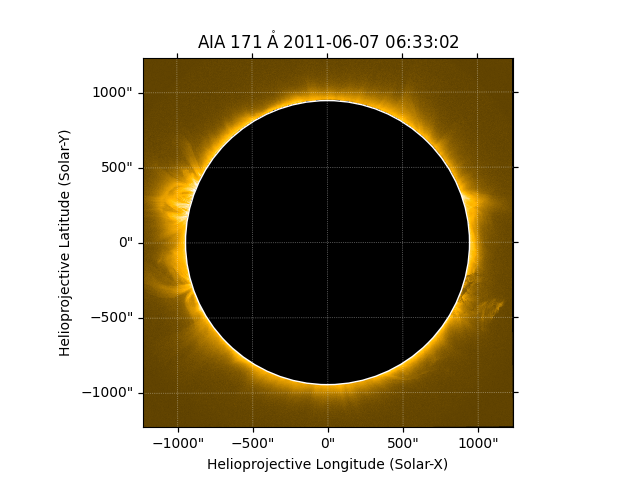# Masking out the solar disk#

How to mask out all emission from the solar disk.

import matplotlib.pyplot as plt

import sunpy.map
from sunpy.data.sample import AIA_171_IMAGE
from sunpy.map.maputils import all_coordinates_from_map, coordinate_is_on_solar_disk


aia = sunpy.map.Map(AIA_171_IMAGE)


A utility function gives us access to the helioprojective coordinate of each pixels. We can use that to create a new array of all the coordinates that are on the solar disk.

hpc_coords = all_coordinates_from_map(aia)


Now, we can create a mask from the coordinates by using another utility function that gives us a mask that has True for those coordinates that are on the solar disk. We also make a slight change to the colormap so that masked values are shown as black instead of the default white.

mask = coordinate_is_on_solar_disk(hpc_coords)
palette = aia.cmap.copy()


Finally we create a new map with our new mask.

scaled_map = sunpy.map.Map(aia.data, aia.meta, mask=mask)


Let’s plot the results using our modified colormap.

fig = plt.figure()